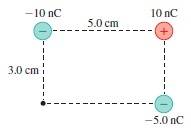# What is the magnitude and direction of the electric field

isukatphysics69

## Homework Statement

What is the magnitude and direction of the electric field at the position indicated by the dot in the figure below? Give your answer in component form in the blanks below.What is the x-component of the electric field at the indicated point?

kq/r^2

## The Attempt at a Solution

Electric field from 1 = [(8.99x10^9)(-10x10^-9)]/((0.03)^2) = -99888.8 Nc
Electric field from 2 = [(8.99x10^9)(10x10^-9)]/((0.05830951895)^2) =26441.17 Nc
Electric field from 3 = [(8.99x10^9)(-10x-5^-9)]/(0.05^2) = -17980 Nc

Total magnitude of the net electric field = sqrt((-99888.8)^2+(26441.17)^2+(-17980)^2) = 104881.8685 Nc

Now I am confused as to how to find my angle for the direction at the point in the picture. there are three different particles having an effect, if there where one id know how to find the correct angle to find the x component, but I am unsure how to get my angle here

#### Attachments

isukatphysics69
I added up the vectors graphically and found the magnitude and got an angle of 252 degrees in quadrant 3. does this look correct?

Mentor
Electric field from 1 = [(8.99x10^9)(-10x10^-9)]/((0.03)^2) = -99888.8 Nc
Electric field from 2 = [(8.99x10^9)(10x10^-9)]/((0.05830951895)^2) =26441.17 Nc
Electric field from 3 = [(8.99x10^9)(-10x-5^-9)]/(0.05^2) = -17980 Nc
Each of these field contributions has a direction. See if you can figure out each one's x and y component.

•isukatphysics69
isukatphysics69
Each of these field contributions has a direction. See if you can figure out each one's x and y component.
hey please see above, I added the vectors graphically on graphing paper and got 252 degrees
Each of these field contributions has a direction. See if you can figure out each one's x and y component.
So I am imagining a unit circle around the point, charge 1 has an angle of 90 degrees, charge 2 has an angle of arctan(3/5) = 30 degrees and charge 3 has an angle of 0 degrees, but then how do I find the angle on the particle?

Mentor
Try this: The field from the -10 nC charge (at the point in question) acts in what direction? What would be its x and y components?

•isukatphysics69
isukatphysics69
Try this: The field from the -10 nC charge (at the point in question) acts in what direction? What would be its x and y components?
The x component would be cos(270) = 0 and the y component would be sin(270) = -1

Mentor
The x component would be cos(270) = 0 and the y component would be sin(270) = -1
Almost: Tell me in words which direction that field points.

isukatphysics69
Almost: Tell me in words which direction that field points.
the direction of the field from charge 1 onto point P points straight down

Mentor
the direction of the field from charge 1 onto point P points straight down
Why down? (The charge is negative.)

•isukatphysics69
isukatphysics69
Why down? (The charge is negative.)
am I supposed to assume that the test charge is positive?? then up. wasn't sure about what sign the test charge was

Mentor
am I supposed to assume that the test charge is positive?? then up. wasn't sure about what sign the test charge was
No test charge is needed or mentioned. You're finding the field at a point.

•isukatphysics69
isukatphysics69
No test charge is needed or mentioned. You're finding the field at a point.
oh god I am confused now

Mentor
Hint: The field from a positive charge points in which direction: Towards or away from the charge?

•isukatphysics69
isukatphysics69
I thought you need a test charge to find the field at a point

isukatphysics69
Hint: The field from a positive charge points in which direction: Towards or away from the charge?
away

Mentor
I thought you need a test charge to find the field at a point
Nope. (But it can be useful to imagine one there.)

away
Good. And for a negative charge?

•isukatphysics69
isukatphysics69
Nope. (But it can be useful to imagine one there.)

Good. And for a negative charge?
towards, so it would be pointed upwards

Mentor
towards, so it would be pointed upwards
Exactly. So the y-component would be what?

Now do the same thinking for the other charges.

•isukatphysics69
isukatphysics69
Exactly. So the y-component would be what?

Now do the same thinking for the other charges.
so the y component would be 99888.8sin(90) = 99888.8 Nc

isukatphysics69
oh so I just sum the components

isukatphysics69
So do you agree the net component for x is -4693 nC

isukatphysics69
YES I GOT IT!

isukatphysics69
I got 1.62/2 points isn't the unit nanocolumn?

Mentor
I got 1.62/2 points isn't the unit nanocolumn?
I haven't checked your numbers. The proper unit for electric field is N/C (Newtons per Coulomb).

•isukatphysics69